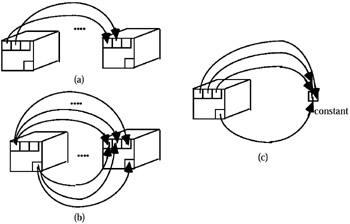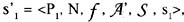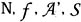# COMPARISON

This operator was proposed for the first time (and in a simpler form) in Fortunato et al. (1986), and consists of a family of operators.

The original proposal was subsequently enlarged, in order to distinguish between the T-COMPARISON (where T means table, see Figure 17(a) and 17(b)) and N-COMPARISON (where N means single numeric constant, see Figure 17(c)).Figure 17

The former compares the MAD in input with another MAD (table), which can be the same, opportunely shifted along a dimension.

The latter compares each measure instance of the MAD in input with a single numeric value. Both these comparisons are performed by a set θ = {>, , <, , =, } of operators.

The comparison between two MADs can be performed in two ways: 1) comparing between the values which are inside the two corresponding cells of the two MADs; 2) or comparing the value inside the first cell of the first MAD with all the measure values of the second MAD, the second cell of the first MAD with all the measure values of the second MAD, and so on, until the last cell of the first MAD (compared, like the previous ones, with all the measure values of the second MAD). The former is denoted by T1:1-Comparison, the latter by T1:n-Comparison.

By this operator we are able to answer queries of the type:

1. In which region has the production of cereals been greater than the previous year?

2. In which region has the production of cereals been greater than "100 tons?"

The result of this operation can be of two types: logical (i.e., in every cell of the resulting MAD, one logic value—true or false—appears), or numerical (if the Ø-condition is verified, value = true, the original value remains inside the cell, otherwise the symbol — appears.)

The result of this operation between s1 and s2 consists of a new MADwhereare the same of s1 and p1 is the new name of the MAD;

s1 has its instance equal to the corresponding instance of s if the comparison verifies the θ-condition (where θ is one of the operator <>, , <, , =, ), while it has another instance equal to "" (null value) if the θ-condition is not verified.

Obviously, depending on the type of comparison considered, the term to the corresponding instance of is substituted by the term to the instance of or with the term to the number.Multidimensional Databases: Problems and Solutions
ISBN: 1591400538
EAN: 2147483647
Year: 2003
Pages: 150